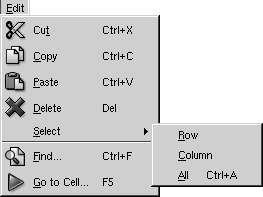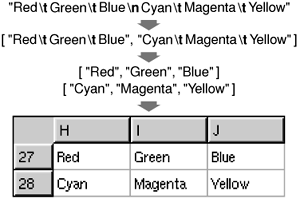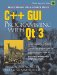We are now ready to implement the slots that correspond to the application's Edit menu.

```void Spreadsheet::cut()
{
copy();
del();
}
```

The cut() slot corresponds to Edit|Cut. The implementation is simple since Cut is the same as Copy followed by Delete.```void Spreadsheet::copy()
{
QTableSelection sel = selection();
QString str;

for (int i = 0; i < sel.numRows(); ++i) {
if (i > 0)
str += "
";
for (int j = 0; j < sel.numCols(); ++j) {
if (j > 0)
str += "	";
str += formula(sel.topRow() + i, sel.leftCol() + j);
}
}
QApplication::clipboard()->setText(str);
}
```

The copy() slot corresponds to Edit|Copy. It iterates over the current selection. Each selected cell's formula is added to a QString, with rows separated by newline characters and columns separated by tab characters.

Figure 4.7. Copying a selection onto the clipboardThe system clipboard is available in Qt through the QApplication::clipboard() static function. By calling QClipboard::setText(), we make the text available on the clipboard, both to this application and to other applications that support plain text. Our format with tab and newline characters as separator is understood by a variety of applications, including Microsoft Excel.

```QTableSelection Spreadsheet::selection()
{
if (QTable::selection(0).isEmpty())
return QTableSelection(currentRow(), currentColumn(),
currentRow(), currentColumn());
return QTable::selection(0);
}
```

The selection() private function returns the current selection. It depends on QTable::selection(), which returns a selection by number. Since we set the selection mode to Single, there is only one selection, numbered 0. But it's also possible that there is no selection at all. This is because QTable doesn't treat the current cell as a selection in its own right. This behavior is reasonable, but slightly inconvenient here, so we implement a selection() function that either returns the current selection or, if there isn't one, the current cell.

```void Spreadsheet::paste()
{
QTableSelection sel = selection();
QString str = QApplication::clipboard()->text();
QStringList rows = QStringList::split("
", str, true);
int numRows = rows.size();
int numCols = rows.first().contains("	") + 1;

if (sel.numRows() * sel.numCols() != 1
&& (sel.numRows() != numRows
|| sel.numCols() != numCols)) {
tr("The information cannot be pasted because the "
"copy and paste areas aren't the same size."));
return;
}

for (int i = 0; i < numRows; ++i) {
QStringList cols = QStringList::split("	", rows[i], true);
for (int j = 0; j < numCols; ++j) {
int row = sel.topRow() + i;
int col = sel.leftCol() + j;
if (row < NumRows && col < NumCols)
setFormula(row, col, cols[j]);
}
}
somethingChanged();
}
```

The paste() slot corresponds to Edit|Paste. We fetch the text on the clipboard and call the static function QStringList::split() to break the string into a QStringList. Each row becomes one string in the QStringList.

Next, we determine the dimension of the copy area. The number of rows is the number of strings in the QStringList; the number of columns is the number of tab characters in the first row, plus 1.

If only one cell is selected, we use that cell as the top-left corner of the paste area. Otherwise, we use the current selection as the paste area.

To perform the paste, we iterate over the rows and split each of them into cells by using QStringList::split() again, but this time using tab as the separator. Figure 4.8 illustrates the steps.

Figure 4.8. Pasting clipboard text into the spreadsheet```void Spreadsheet::del()
{
QTableSelection sel = selection();
for (int i = 0; i < sel.numRows(); ++i) {
for (int j = 0; j < sel.numCols(); ++j)
delete cell(sel.topRow() + i, sel.leftCol() + j);
}
clearSelection();
}
```

The del() slot corresponds to Edit|Delete. It is sufficient to use delete on each of the Cell objects in the selection to clear the cells. The QTable notices when its QTableItems are deleted and automatically repaints itself. If we call cell() with the location of a deleted cell, it will return a null pointer.

```void Spreadsheet::selectRow()
{
clearSelection();
QTable::selectRow(currentRow());
}

{
clearSelection();
QTable::selectColumn(currentColumn());
}

{
clearSelection();
selectCells(0, 0, NumRows  1, NumCols  1);
}
```

The selectRow(), selectColumn(), and selectAll() functions correspond to the Edit|Select|Row, Edit|Select|Column, and Edit|Select|All menu options. The implementation relies on QTable's selectRow(), selectColumn(), and selectCells() functions.

```void Spreadsheet::findNext(const QString &str, bool caseSensitive)
{
int row = currentRow();
int col = currentColumn() + 1;

while (row < NumRows) {
while (col < NumCols) {
if (text(row, col).contains(str, caseSensitive)) {
clearSelection();
setCurrentCell(row, col);
setActiveWindow();
return;
}
++col;
}
col = 0;
++row;
}
qApp->beep();
}
```

The findNext() slot iterates through the cells starting from the cell to the right of the cursor and moving right until the last column is reached, then continues from the first column in the row below, and so on until the text is found or until the very last cell is reached. For example, if the current cell is cell C27, we search D27, E27, ..., Z27, then A28, B28, C28, ..., Z28, and so on until Z999. If we find a match, we clear the current selection, we move the cell cursor to the cell that matched, and we make the window that contains the Spreadsheet active. If no match is found, we make the application beep to indicate that the search finished unsuccessfully.

```void Spreadsheet::findPrev(const QString &str, bool caseSensitive)
{
int row = currentRow();
int col = currentColumn()  1;

while (row >= 0) {
while (col >= 0) {
if (text(row, col).contains(str, caseSensitive)) {
clearSelection();
setCurrentCell(row, col);
setActiveWindow();
return;
}
col;
}
col = NumCols  1;
row;
}
qApp->beep();
}
```

The findPrev() slot is similar to findNext(), except that it iterates backward and stops at cell A1.C++ GUI Programming with Qt 3
ISBN: 0131240722
EAN: 2147483647
Year: 2006
Pages: 140